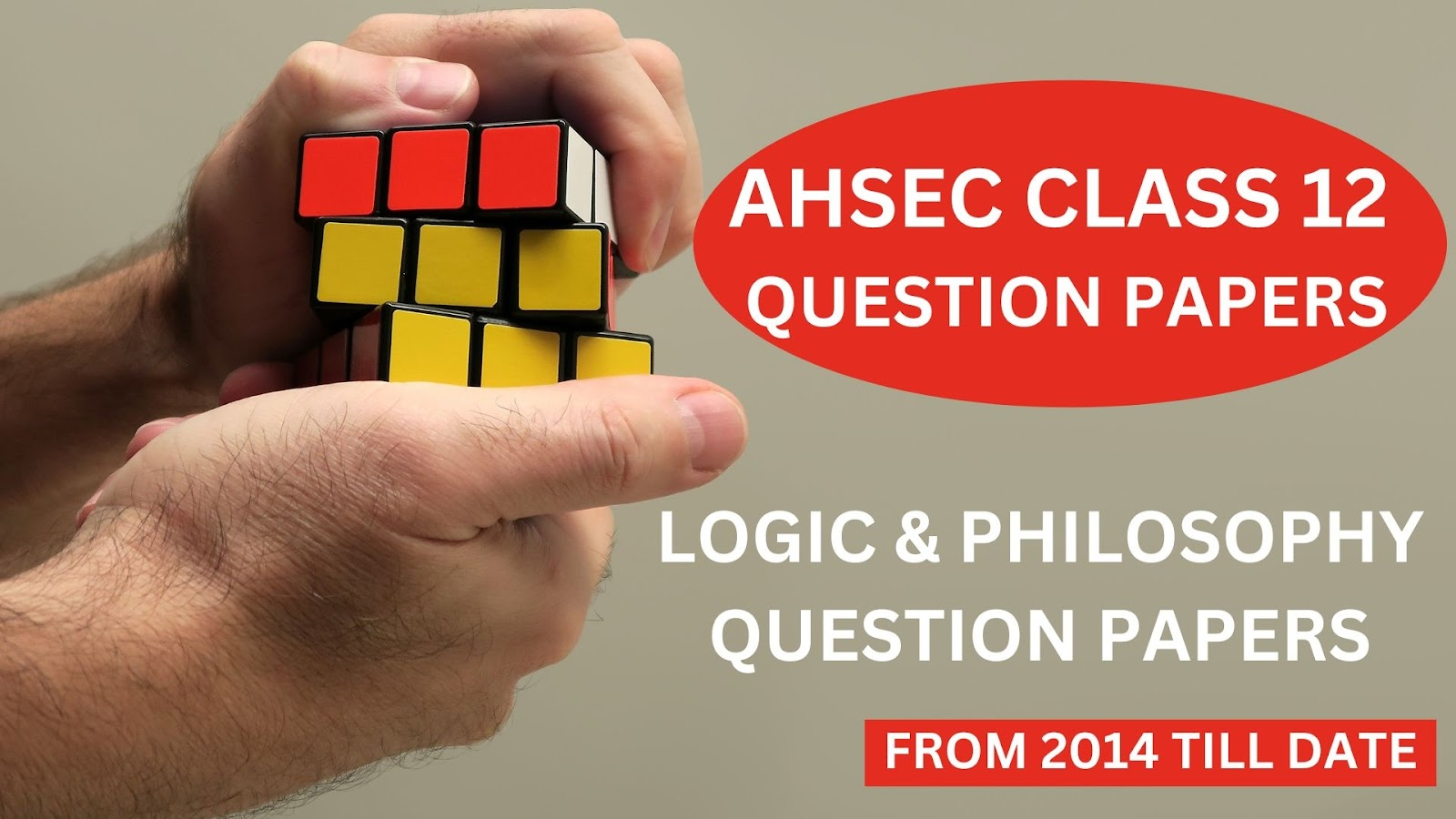# Logic and Philosophy Question Paper' 2017 [AHSEC Class 12 Question Papers]## Logic and Philosophy Question Paper' 2017AHSEC Class 12 Question PapersFull Marks: 100Pass Marks: 30Time: 3 hours

The figures in the margin indicate full marks for the questions.
1. Give very short answer:                                           1x12=12
a)      What is the essence of induction proper?
b)      Is perfect induction an induction proper?
c)       What is the ground of Analogy?
d)      “A cause is the sum-total of conditions positive and negative taken together” – Who said the statement?
e)      “The law of causation is the material ground of induction” – is it true?
f)       What is ‘Vera Causa’?
g)      What is the meaning of the Latin word ‘Mores’?
h)      Who is the founder of scientific realism?
i)        “Observation is finding a fact and experiment is making one” – Who said this?
j)        How many Purusarthas are there?
k)      Is ethics a positive science?
l)        From which Sanskrit root the word ‘Dharma’ is derived?
2. Define scientific induction?                     2
3. Explain very briefly why the conclusion of unscientific induction is probable.                   2
4. Why observation and experiment are called the material grounds of induction?                            2
5. State two advantages of simple observation.                                                 2
Or
What do you mean by ‘Paradox of Induction’?                                               2
6. Mention two features of scientific realism.                                     2
7. Define primary qualities with examples.                                           2
8. What is the meaning of ‘esse est percipii’?                                      2
9. Name the form of idealism with which dialectical method is associated. State the name of the philosopher who advocated this method.                                                2
10. State two features of objective idealism.                                       2
11. Why is habitual action considered as an object of moral judgement?                                              2
12. State the second canon of elimination. Name the experimental method which is based on this cannon of elimination.                                 1+1=2
Or
State one advantage of the method of Agreement. Why is the method called the method of discovery?                           1+1
13. State two points of difference between inductive and deductive inference.                                 2
14. Explain the meaning of ‘Varnasrama dharma’.                                             4
15. State Flint’s definition of religion.                      4
Or
Mention any four characteristics of religion.                                                    4
16. Mention two practical imperfections of the material of Agreement.                                4
17. State two points of similarity between religion and morality.                            2+2=4
Or
State four points about the nature of hypothesis.                                        4
18. Distinguish between good analogy and bad analogy.                                                4
Or
Distinguish between cause and condition.                                                        4
19. State along with brief explanations about the four conditions of legitimate hypothesis.           4
20. Write short note on the fallacy of post hoc ergo propter hoc.                                               4
21. Name the theory which states that external world can be known directly as it really is. Also, define this theory and state one important drawback of this theory.                          1+2+1=4
22. Write short note on “conflict of desires”.                                                       4
Or
What is voluntary action? State the three stages of voluntary action.                     1+3=4
23. Define Berkeley’s Subjective Idealism. Is Berkeley a solipsist?                           2+2=4
24. Define unscientific induction with example. State why is it called ‘Unscientific’? Mention briefly three characteristics of unscientific induction.      2+1+3=6